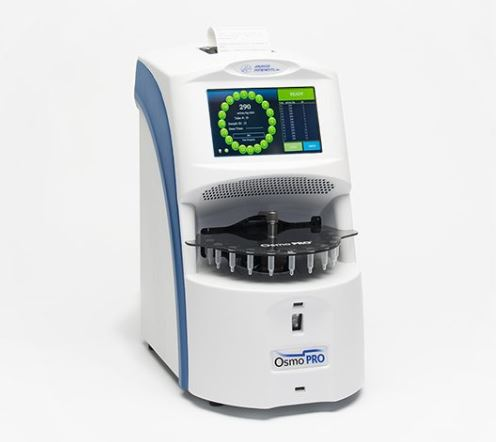# Comparing the conductivity of two different solutionsConductivity is perhaps the most misunderstood and erroneously used measurement in hydroponic culture. This has a lot to do with conductivity also being called a “totally dissolved solid” (TDS) measurement and the conductivity scale being expressed in “ppm” units, concentration units which only cause confusion in this area. Today I want to talk about an important consequence of this confusion that happens when you try to compare the conductivity of different nutrient solutions. I’ll talk about a recent case I encountered and how it generated significant problems due to a natural misunderstanding of how conductivity works.A grower wanted to run a side by side trial of two nutrient formulations using identical growing conditions. This grower then decided that the best way to do this was to ensure that the conductivity and pH of the two solutions were identical after preparing the nutrient solutions, then they would both be equivalent in terms of their strength and differences in results would be entirely due to the differences in ionic ratios between both of them. The media was the same, the environment was the same and plant genetics were the same.

However there was a small problem with this thinking. The same conductivity across two different solutions is not the same thing. You might think that using a conductivity of 2.0 mS/cm across two different nutrient solutions might mean that their “strength” is the same, but in reality the strength of a solution – as per what a plant really experiences – is determined by its osmotic pressure and osmotic pressure – although proportional to conductivity within the same solution – cannot be extrapolated when the composition of the solution changes. This confusion is further expanded when people see the conductivity numbers in ppm because the expression in mg/L makes them think there is the same “amount of stuff” in the two solutions. This is not the case.

All the ppm does is tell you that your solution has the same conductivity as a reference with that ppm concentration (commonly NaCl or KCl) but it tells you nothing about how many dissolved solids are really present within your nutrient solution. Given that non-conductive substances also affect the osmotic pressure of a solution it can happen that a nutrient solution with the same conductivity as another one in reality has a lot more dissolved solids, making it far more concentrated in real terms compared to the other one.In the above mentioned particular case one solution had a chelating agent that effectively made a significant number of ions neutral in charge (effectively making them non-conductive) reducing the measured conductivity by around 20% at the same osmotic pressure as the other solution. So while the grower was feeding the two solutions at the exact same conductivity, the second solution was around 20% more concentrated in real terms – osmotic pressure terms – compared to the other one. Plants responded very negatively to this – as the conductivity was already quite high – so the grower erroneously assumed that this was due to the ionic ratios instead of it simply being due to an error in judging concentrations. The second solution was a lot stronger in real terms, although the conductivity was the same.

When comparing two nutrient solutions you should therefore resort to measurements different than conductivity because the conductivity of two different solutions with different ion compositions cannot be compared, the same level of conductivity will result in two completely different osmotic pressure values. Their strengths will not be the same. If you want to compare two different solutions at the same real strength then you need to use an osmometer to determine this point and sadly osmometers are neither cheap nor practical to use.

However another possibility is to simply compare at a constant concentration of a given element. Have a lab analysis of the two fertilizers made – remember you cannot trust labels to give you the real composition values – calculate how much of a given element, for example N, is present at a given application rate and then dial in the other fertilizer to match that N concentration. The osmotic pressures will probably be different but at least under this sort of A/B test you will be comparing apples to apples in the sense that the only variable will be the N:X ionic ratios between the two solutions. Total strengths will differ but this will be due to differences in ionic ratios, which is probably what you want to test.

### 1 Comment

• […] yet a very poorly understood one. I have written several posts about conductivity in the past (1,2,3) and today I want to talk about the use of the term “Total Dissolved Solids” and the […]

Subscribe Today!